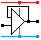Home

rOm
Quest
Glossary

Random
Page
Search
Site
Lush
Sim
Class
Subject
Images

Help
FAQ
Sign
Up
Log
In
 AS Level     Semiconductor     Diode     Rectifier and PSU     >Zener Diode<

# Semiconductor Zener Diode

Site for Eduqas/WJEC - Go to the AQA site.

AS Level    Semiconductors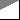Questions 0 to 4   -->  View All

Zener diodes are used to provide an accurate reference voltage. They "break down" at a specified voltage in "reverse bias".

Zener Diode in the Lushprojects Simulator    Slide the resistance slider to simulate different load currents. The 4 V Zener level does not change.

## Zener Diode Properties

All diodes allow current to pass in one direction only. In normal use, diodes never suffer a reverse breakdown which would destroy the diode.

Zener Diodes are special because they can break down without being damaged. They do this at a specific voltage (the Zener voltage) which is accurately determined by the manufacturing process. It is possible to purchase Zener diodes with a range of breakdown voltages between about 3.3 up to 200 volts.

• Zener diodes are connected the opposite way round to normal diodes (REVERSE BIASED).
• 5 or more mA reverse breakdown current is required to flow through the Zener diode to ensure the Zener voltage is reliably established. The exact figure is quoted in the data sheet for the diode.
• Once 5 or more mA are flowing , the current through the diode can increase without the voltage across the diode increasing at all. In this way, the Zener diode can provide a stable reference voltage.

## Zener Diode Calculations - AS and A Level• The resistor ( R ) voltage is 9 - 4 Volts. This is needed when you calculate the resistance of R.
• The Zener diode voltage is 4 Volts ( in this example ).
• Assume the load takes a maximum current of 95 mA.
• For reliable operation, the Zener diode must take at least 5 mA.
• Under maximum load, the total current will be 100 mA.
• If the load current decreases, the Zener current increases by the exact same amount.
• With the load disconnected, the Zener current rises to 100mA.
• This behaviour ensures that the voltage across the Zener diode remains constant.

## Resistor Calculation

If the Zener voltage is 4V and the supply voltage is 9V, the potential difference across R will be 5V.

Using Ohm's Law ...

• R = V / I
• R = (9 - 4) / (100 x 10-3)
• R = 50Ω
• The nearest suitable preferred value is 47Ω
• 51Ω would not be suitable because the Zener current might drop below 5mA.

## Zener Diode Power Rating

To calculate the Zener diode power rating ...

Power_Rating = (Maximum_Load_Current + Minimum_Zener_Current) x Zener_Breakdown_Voltage

P = (95 x 10-3 + 5 x 10-3) x 4

P = 400mW or 0.4W

## Zener Diode Power Calculation

Power = I V        Power = I2 R        Power = V2 / R

This diagram shows a Zener diode operating under typical conditions. In exam questions, the diode is often operating under it's extreme conditions with either zero or maximum load current. In all cases, the total circuit current is the same.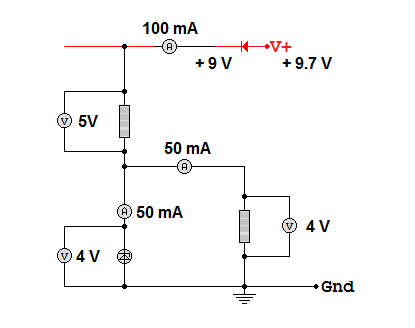P = I V        P = 0.05 x 4        P = 0.2 Watts

Resistor Power

Use the resistor voltage and the resistor current.

P = I V        P = 0.1 x 5        P = 0.5 Watts

Zener Diode Power

Use the Zener diode voltage and the Zener diode current.

P = I V        P = 0.05 x 4        P = 0.2 Watts

Whole Circuit Power

Use the whole circuit voltage and the whole circuit current.

P = I V        P = 0.1 x 9        P = 0.9 Watts

Notice how all the separate component powers add up to the whole circuit power.

## Zener Diode Characteristics

The graph below shows the current/voltage characteristics of a Zener diode. The reverse leakage current has been exaggerated to make it show on the graph. The reverse breakdown voltage varies between different Zener diodes. The range of values goes from a few Volts up to about 200 Volts.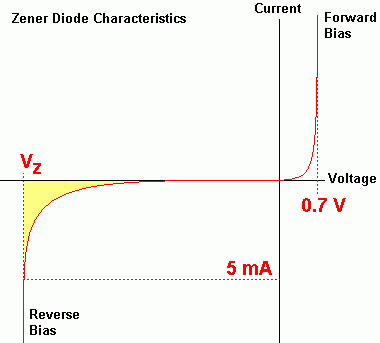If the reverse bias current is less than about 5mA, the Zener voltage will be lower than the expected value. When designing circuits to give a reference voltage using a Zener diode, the minimum Zener current should always be at least 5 mA (check the data sheet). The yellow region in the graph shows where the Zener voltage is not properly established.

## Practical Task - LED Characteristics

This circuit can be used to test the behaviour of any low voltage / low current diode. These include typical silicon diodes such as the 1N4001, LEDs and Zener diodes. The activity below shows an LED.

Build this circuit and measure and record the potential difference across the diode and the current through it. Repeat these measurements for the full range of movement of the potentiometer. Plot a graph of your results. Plot your graph as you go and get sufficient readings before you dismantle your circuit.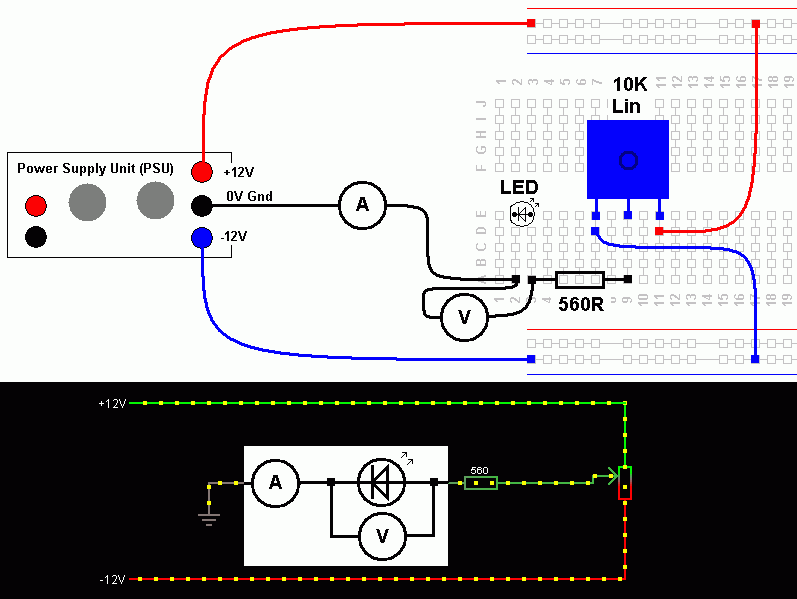• Take all the necessary measurements - do this before you dismantle your circuit.
• Plot a graph showing the current through a diode as the potential difference across it is altered.
• Take additional measurements to fill gaps in your graph - especially where the graph is curved.

Make sure there are no gaps in your graph where extra measurements should have been taken.

Subject Name     Level     Topic Name     Question Heading     First Name Last Name Class ID     User ID

 CloseEscapeX Q: qNum of last_q     Q ID: Question ID         Score: num correct/num attempts         Date Done

Question Text

image url

Help Text
Debug

• You can attempt a question as many times as you like.
• If you are logged in, your first attempt, each day, is logged.
• To improve your scores, come back on future days, log in and re-do the questions that caused you problems.
• In the grade book, you can delete your answers for a topic before re-doing the questions. Avoid deleting unless you intend re-doing the questions very soon.